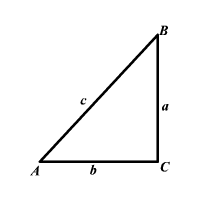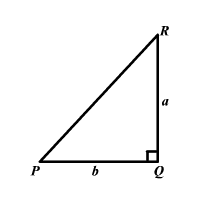# The Converse of Pythagorean Theorem

We assume you're familiar with the Pythagorean Theorem.

The converse of the Pythagorean Theorem is:

If the square of the length of the longest side of a triangle is equal to the sum of the squares of the other two sides, then the triangle is a right triangle.

That is, in $\Delta ABC$, if ${c}^{2}={a}^{2}+{b}^{2}$ then $\angle C$ is a right triangle, $\Delta PQR$ being the right angle.We can prove this by contradiction.

Let us assume that ${c}^{2}={a}^{2}+{b}^{2}$ in $\Delta ABC$ and the triangle is not a right triangle.

Now consider another triangle $\Delta PQR$. We construct $\Delta PQR$ so that $PR=a$, $QR=b$ and $\angle R$ is a right angle.By the Pythagorean Theorem, ${\left(PQ\right)}^{2}={a}^{2}+{b}^{2}$.

But we know that ${a}^{2}+{b}^{2}={c}^{2}$ and ${a}^{2}+{b}^{2}={c}^{2}$ and $c=AB$.

So, ${\left(PQ\right)}^{2}={a}^{2}+{b}^{2}={\left(AB\right)}^{2}$.

That is, ${\left(PQ\right)}^{2}={\left(AB\right)}^{2}$.

Since $PQ$ and $AB$ are lengths of sides, we can take positive square roots.

$PQ=AB$

That is, all the three sides of $\Delta PQR$ are congruent to the three sides of $\Delta ABC$. So, the two triangles are congruent by the Side-Side-Side Congruence Property.

Since $\Delta ABC$ is congruent to $\Delta PQR$ and $\Delta PQR$ is a right triangle, $\Delta ABC$ must also be a right triangle.

This is a contradiction. Therefore, our assumption must be wrong.

Example 1:

Check whether a triangle with side lengths $6$ cm, $10$ cm, and $8$ cm is a right triangle.

Check whether the square of the length of the longest side is the sum of the squares of the other two sides.

$\begin{array}{l}{\left(10\right)}^{2}\stackrel{?}{=}{\left(8\right)}^{2}+{\left(6\right)}^{2}\\ \text{\hspace{0.17em}}\text{\hspace{0.17em}}100\stackrel{?}{=}64+36\\ \text{\hspace{0.17em}}100=100\end{array}$

Apply the converse of Pythagorean Theorem.

Since the square of the length of the longest side is the sum of the squares of the other two sides, by the converse of the Pythagorean Theorem, the triangle is a right triangle.

A corollary to the theorem categorizes triangles in to acute, right, or obtuse.

In a triangle with side lengths $a$, $b$, and $c$ where $c$ is the length of the longest side,

if ${c}^{2}<{a}^{2}+{b}^{2}$ then the triangle is acute, and

if ${c}^{2}>{a}^{2}+{b}^{2}$ then the triangle is obtuse.

Example 2:

Check whether the triangle with the side lengths $5$, $7$, and $9$ units is an acute, right, or obtuse triangle.

The longest side of the triangle has a length of $9$ units.

Compare the square of the length of the longest side and the sum of squares of the other two sides.

Square of the length of the longest side is ${9}^{2}=81$ sq. units.

Sum of the squares of the other two sides is

That is, ${9}^{2}>{5}^{2}+{7}^{2}$.

Therefore, by the corollary to the converse of Pythagorean Theorem, the triangle is an obtuse triangle.